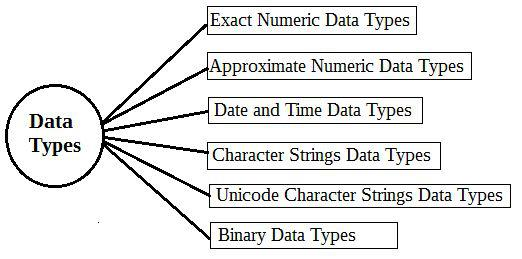Loading, please wait...## Data Types

How To Use Data Types In SQL

Introduction:

In this article, you will learn, how to use Data Types in SQL.

In SQL, we define the datatype of each data. The datatype is defined in SQL Server when we make the tables. Each datatype is arranged according to the data. In the SQL tables, it is compulsory to give the datatype of every item. Datatypes are not only used in the SQL. It is used in every database like- Java, My SQL, MS Access etc.

In SQL, we have six types of data types.Exact Numeric Data Types -

• bigint : Its range is from -9,223,372,036,854,775,808 to 9,223,372,036,854,775,807
• int : Its range is from -2,147,483,648 to 2,147,483,647
• smallint : Its range is from -32,768 to 32,767
• tinyint : Its range is from 0 to 255
• bit : Its range is from 0 to 1
• decimal : Its range is from -10^38 +1 to 10^38 -1
• numeric : Its range is from -10^38 +1 to 10^38 -1
• money : Its range is from -922,337,203,685,477.5808 to +922,337,203,685,477.5807
• smallmoney : Its range is from -214,748.3648 to +214,748.3647

Approximate Numeric Data Types -

This data type shows the numeric values.

• float : Its range is from -1.79E + 308 to 1.79E + 308
• real : Its range is from -3.40E + 38 to 3.40E + 38

Date and Time Data Types -

• DateTime: Its range is from Jan 1, 1753, to Dec 31, 9999
• smalldatetime: Its range is from Jan 1, 1900, to Jun 6, 2079
• date: It is used to store a date.

Example: June 22, 2016

• time: It is used to store time.

Example : 2:30 P.M.

Character Strings Data Types -

• char: Its maximum length is of 8,000 characters and the fixed length is non-Unicode data.
• varchar: Its maximum length is of 8,000 characters and variable-length is non-Unicode data.
• varchar(max): Its maximum length is of 231characters and variable-length is non-Unicode data.
• Text: Its maximum length is of 2,147,483,647 characters and the variable length is non-Unicode data.

Unicode Character Strings Data Types -

• nchar: Its maximum length is of 4,000 characters and Fixed-length Unicode.
• nvarchar: Its maximum length is of 4,000 characters and Variable-length Unicode.
• nvarchar(max): Its maximum length is of 231characters and Variable-length Unicode.
• ntext: Its maximum length is of 1,073,741,823 characters Variable-length Unicode.

Binary Data Types

• binary: Its maximum length is of 8,000 bytes and Fixed-length binary data.
• varbinary: Its maximum length is of 8,000 bytes and Variable-length binary data.
• varbinary(max): Its maximum length is of 231 bytes and Variable-length Binary data.
• Image: Its maximum length is of 2,147,483,647 bytes and Variable-length Binary Data

Misc Data Types

• sql_variant: It is used to store the value of the database.
• Timestamp: It is used to store the database values, which updates permanently.
• Uniqueidentifier: It is used for a globally unique identifier.
• Xml: It is used to store XML data in a column.
• Cursor: It performs the reference to an object.
• table: It is used to store the data, which we use easily in future.

Summary:
Thus, we learned that every data has a particular data type in SQL and it defines in SQL when we make tables.